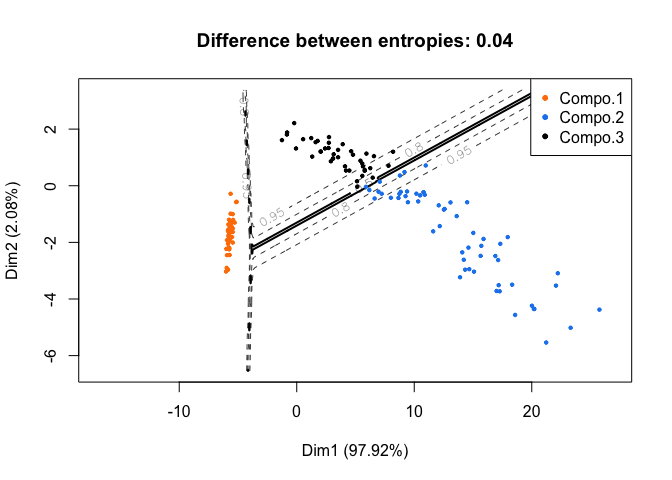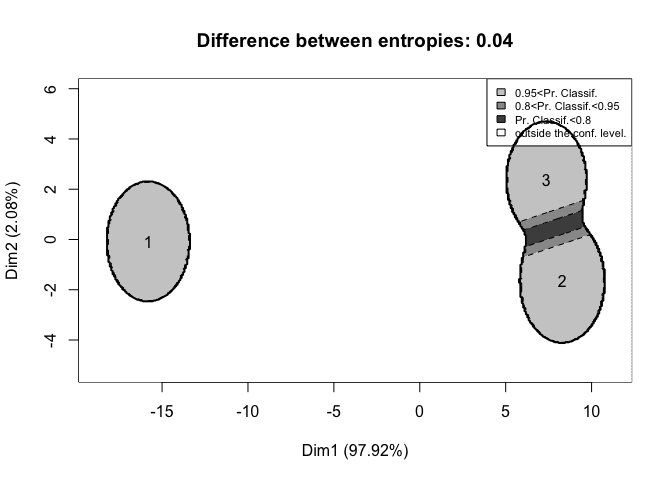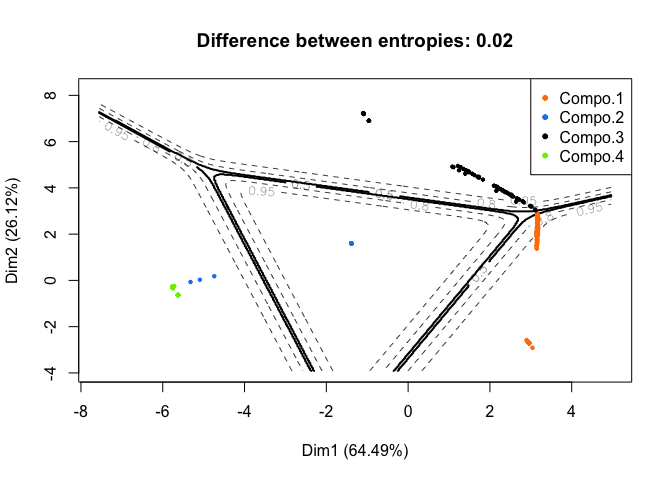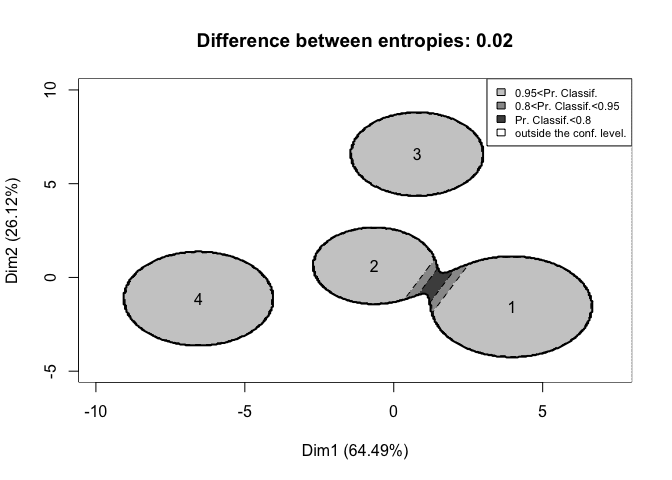# Using ClusVis with RMixtComp Output for Visualization

## ClusVis

ClusVis is an R package that performs a gaussian-based visualization of gaussian and non-gaussian Model-Based Clustering. This visualization is based on the probabilities of classification. See this preprint for more details about the method. It allows to visualize clusters as bivariate spherical gaussian.

## ClusVis and RMixtComp

First, we load the required packages.

library(RMixtComp)
## Loading required package: RMixtCompUtilities
library(ClusVis)

To illustrate the use of ClusVis with RMixtComp output, we use the iris dataset and the congress dataset.

### Example 1: iris dataset

The iris dataset gives the measurements in centimeters of the variables sepal length and width and petal length and width, respectively, for 50 flowers from each of 3 species of iris.

data("iris")
head(iris)
##   Sepal.Length Sepal.Width Petal.Length Petal.Width Species
## 1          5.1         3.5          1.4         0.2  setosa
## 2          4.9         3.0          1.4         0.2  setosa
## 3          4.7         3.2          1.3         0.2  setosa
## 4          4.6         3.1          1.5         0.2  setosa
## 5          5.0         3.6          1.4         0.2  setosa
## 6          5.4         3.9          1.7         0.4  setosa

First, we learn a mixture model with 3 classes for the 4 measurements varaibles.

res <- mixtCompLearn(iris[, -5], nClass = 3, criterion = "BIC", nRun = 3, nCore = 1, verbose = FALSE)

Then, we apply the clusvis function. This function requires 2 parameters: the logarithm of the probabilities of classification of every individuals and the proportion of the mixture.

logTik <- getTik(res, log = TRUE)
prop <- getProportion(res)
resVisu <- clusvis(logTik, prop)

The results can be displayed using the plotDensityClusVisu function. The first graph is generated with the parameter add.obs = TRUE. It overlays on the most discriminative map the curve of iso-probabilities of classification and the cloud of observations.

plotDensityClusVisu(resVisu, add.obs = TRUE)With add.obs = FALSE, the goal of the plot is to represents the overlap between the clusters. Each clusters is represented by its centers and a 95% confidence level border. The differene between entropies displayed in the title defines the accuracy of the representation. A difference closed to 0 means that the representation is accurate.

plotDensityClusVisu(resVisu, add.obs = FALSE)Here, we note that two clusters are closed and so they contains flowers with similar measures whereas the other cluster contains flowers with very different measures from the two others.

### Example 2: congress dataset

This data set includes votes for each of the U.S. House of Representatives Congressmen on the 16 key votes identified by the CQA in 1984.

data("congress")
head(congress)
##           V1 V2 V3 V4 V5 V6 V7 V8 V9 V10 V11 V12 V13 V14 V15 V16 V17
## 1 republican  n  y  n  y  y  y  n  n   n   y   ?   y   y   y   n   y
## 2 republican  n  y  n  y  y  y  n  n   n   n   n   y   y   y   n   ?
## 3   democrat  ?  y  y  ?  y  y  n  n   n   n   y   n   y   y   n   n
## 4   democrat  n  y  y  n  ?  y  n  n   n   n   y   n   y   n   n   y
## 5   democrat  y  y  y  n  y  y  n  n   n   n   y   ?   y   y   y   y
## 6   democrat  n  y  y  n  y  y  n  n   n   n   n   n   y   y   y   y

First, we change the format of the data. The vote “n” is refactored as 1 and “y” as 2. “democrat” is refactored as 1 and “republican” as 2.

## MixtComp Format
congress$V1 = refactorCategorical(congress$V1, c("democrat", "republican", "?"), c(1, 2, "?"))
for(i in 2:ncol(congress))
congress[, i] = refactorCategorical(congress[, i], c("n", "y", "?"), c(1, 2, "?"))

head(congress)
##   V1 V2 V3 V4 V5 V6 V7 V8 V9 V10 V11 V12 V13 V14 V15 V16 V17
## 1  2  1  2  1  2  2  2  1  1   1   2   ?   2   2   2   1   2
## 2  2  1  2  1  2  2  2  1  1   1   1   1   2   2   2   1   ?
## 3  1  ?  2  2  ?  2  2  1  1   1   1   2   1   2   2   1   1
## 4  1  1  2  2  1  ?  2  1  1   1   1   2   1   2   1   1   2
## 5  1  2  2  2  1  2  2  1  1   1   1   2   ?   2   2   2   2
## 6  1  1  2  2  1  2  2  1  1   1   1   1   1   2   2   2   2

We run MixtComp with a Multinomial model for each variable.

model <- rep("Multinomial", ncol(congress))
names(model) = colnames(congress)

res <- mixtCompLearn(congress, model = model, nClass = 4, criterion = "BIC", nRun = 3, nCore = 1)

As before, we extract the required parameters.

logTik <- getTik(res, log = TRUE)
prop <- getProportion(res)
head(logTik)
##           [,1]       [,2]          [,3]          [,4]
## [1,]      -Inf  -6.858874          -Inf -0.0010506472
## [2,]      -Inf  -8.227312          -Inf -0.0002672894
## [3,] -22.30520       -Inf -2.055760e-10          -Inf
## [4,] -10.90311 -15.973071 -1.851677e-05          -Inf
## [5,] -15.90965 -13.566076 -1.406477e-06          -Inf
## [6,] -14.71938 -10.496007 -2.805201e-05          -Inf

It is important to notice that there are a lot of -Inf values in the variable logTik because some probabilities to be in a cluster are exactly 0. If there are too many infinite values, it is a problem for the cluvis function. One way to avoid this problem is to replace infinite values with the logarithm of a epsilon.

logTik[is.infinite(logTik)] = log(1e-20)
head(logTik)
##           [,1]       [,2]          [,3]          [,4]
## [1,] -46.05170  -6.858874 -4.605170e+01 -1.050647e-03
## [2,] -46.05170  -8.227312 -4.605170e+01 -2.672894e-04
## [3,] -22.30520 -46.051702 -2.055760e-10 -4.605170e+01
## [4,] -10.90311 -15.973071 -1.851677e-05 -4.605170e+01
## [5,] -15.90965 -13.566076 -1.406477e-06 -4.605170e+01
## [6,] -14.71938 -10.496007 -2.805201e-05 -4.605170e+01

Now, the clusvis function can be run.

resVisu <- clusvis(logTik, prop)

And the two associated plots generated.

plotDensityClusVisu(resVisu, add.obs = TRUE)plotDensityClusVisu(resVisu, add.obs = FALSE)